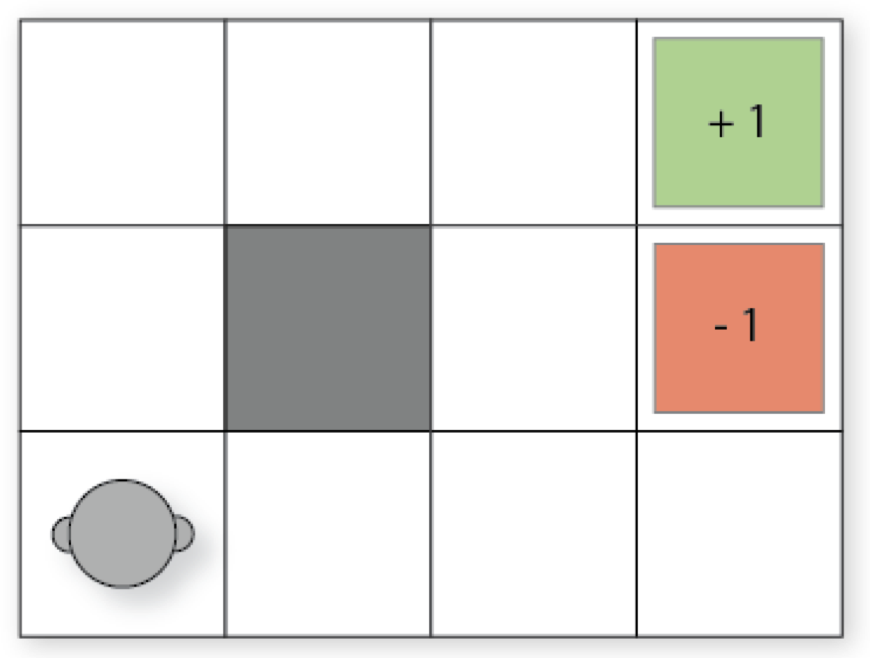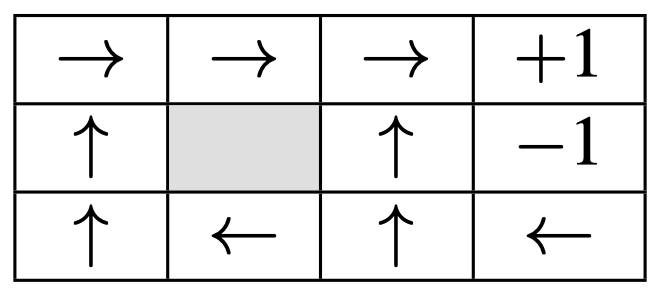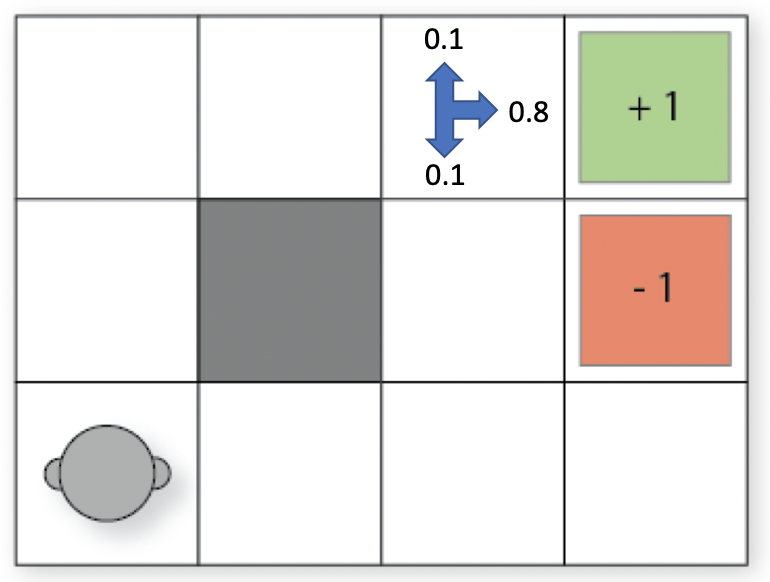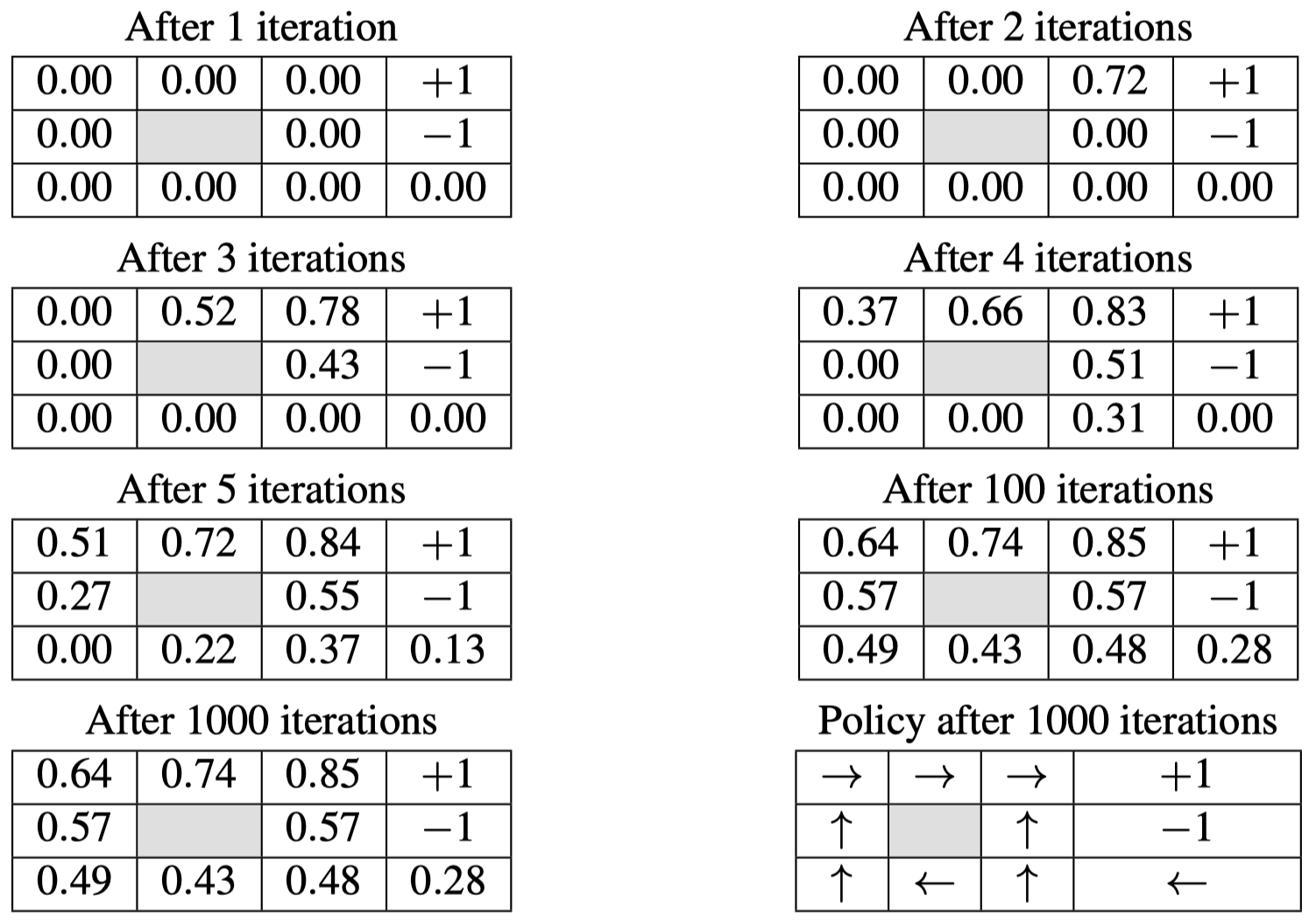# 人工智能自动规划 07：马尔可夫决策过程（MDP）

## 墨尔本大学 COMP90054 课程笔记

Posted by YEY on April 28, 2020

# Lecture 07 马尔可夫决策过程（MDP）

1. 动机
2. 马尔可夫决策过程（MDP）：定义
3. 计算：求解 MDP
4. 部分可观察 MDP

## 1. 动机

### 1.1 学习成果

1. 确定在哪些情况下，马尔可夫决策过程（MDP）模型适用于我们的问题。
2. 定义 “马尔可夫决策过程”。
3. 比较 MDP 与经典规划模型。
4. 说明 Bellman 方程（和动态规划）是如何求解 MDP 问题的。
5. 应用价值迭代来手动解决小规模 MDP 问题，并编写价值迭代算法的代码来自动解决中等规模的 MDP 问题。
6. 根据价值函数构造策略。
7. 比较和对比价值迭代与策略迭代。
8. 讨论价值迭代和策略迭代算法的优缺点。

### 1.2 相关阅读

• 任何概率论基础材料。
• Chapter 17 of Artiﬁcial Intelligence — A Modern Approach by Russell and Norvig.
• Chapter 4 of Reinforcement Learning: An Introduction, second edition.

### 1.3 移除假设

• 确定性事件
• 环境改变只是行动导致的结果
• 完美的知识（全知）
• 单个执行器（全能）

### 1.4 马尔可夫决策过程

• 抛一枚硬币有 2 种结果：正面（$\frac{1}{2}$）和背面（$\frac{1}{2}$）
• 同时掷两个骰子有 12 种结果：2（$\frac{1}{36}$）, 3（$\frac{2}{36}$）, 4（$\frac{3}{36}$）, $\dots$, 12（$\frac{1}{36}$）
• 当试图用一个机械臂拾取物体时，可以有 2 种结果：成功（$\frac{4}{5}$）和失败（$\frac{1}{5}$）

MDP 已成功应用于许多领域的规划中：机器人导航、规划在矿山的哪个区域挖掘矿物、为患者提供治疗、对车辆进行维护计划等。

### 1.5 典型例子：网格世界• 如果 agent 尝试向北移动，我们假设有 $80\%$ 的时间（即 $0.8$ 的概率）它会按照计划移动（假设途中没有墙）
• 有 $10\%$ 的时间（即 $0.1$ 的概率），试图向北移动会导致 agent 向西移动（假设途中没有墙）
• 有 $10\%$ 的时间（即 $0.1$ 的概率），试图向北移动会导致 agent 向东移动（假设途中没有墙）
• 如果碰到墙，则 agent 会停在原地。

## 2. 马尔可夫决策过程（MDP）：定义

### 2.1 折扣回报马尔可夫决策过程（Discounted Reward MDP）

MDP 是 完全可观测的、概率的 状态模型。MDP 的最常见表述是 折扣回报 马尔可夫决策过程：

• 一个状态空间 $S$
• 初始状态 $s_0\in S$
• 行动 $A(s)\subseteq A$ 可应用于每个状态 $s\in S$
• 转移概率 $P_a(s’\mid s)$，对于 $s\in S$ 和 $a\in A(s)$
• 回报 $r(s,a,s’)$ 可以为正或负，采取行动 $a$ 从状态 $s$ 转换为状态 $s’$
• 一个 折扣因子 $0\le \gamma <1$

• 转移函数不再是确定性的。每个行动有 $P_a(s’\mid s)$ 的概率达到状态 $s’$，如果行动 $a$ 执行之前的状态为 $s$。
• 没有目标。每个行动在采取之后都会接受一个回报。回报的值取决于其采用的状态。
• 没有行动代价，它们被建模为负的回报。
• 我们有一个折扣因子。

MDP 可以视为经典规划的一种扩展，它使得我们可以模拟更多的问题，但是也会使得求解过程和经典规划非常不一样。

### 2.2 折扣回报

\begin{align} V &= r_1+\gamma r_2 + \gamma^2 r_3 +\gamma^3 r_4 + \dots \\ &= r_1 + \gamma(r_2+\gamma(r_3+\gamma(r_4+\dots))) \end{align}

$V = r_1+r_2 + r_3 + r_4 + \dots$

### 2.3 MDP 建模：概率 PDDL

1
2
3
4
5
6
7
8
(define (domain bomb-and-toilet)
(:requirements :conditional-effects :probabilistic-effects)
(:predicates (bomb-in-package ?pkg) (toilet-clogged) (bomb-defused))

(:action dunk-package
:parameters (?pkg)
:effect (and (when (bomb-in-package ?pkg) (bomb-defused))
(probabilistic 0.05 (toilet-clogged))))


### 2.4 MDP 问题的解：策略$[\texttt{move_up},\texttt{move_up},\texttt{move_right}, \texttt{move_right}, \texttt{move_right}]$• $at(0,0) \; \Longrightarrow \; \texttt{move_up}$
• $at(0,1) \; \Longrightarrow \; \texttt{move_up}$
• $at(0,2) \; \Longrightarrow \; \texttt{move_right}$
• $at(1,0) \; \Longrightarrow \; \texttt{move_left}$
• $at(1,2) \; \Longrightarrow \; \texttt{move_right}$
• $at(2,0) \; \Longrightarrow \; \texttt{move_up}$
• $at(2,1) \; \Longrightarrow \; \texttt{move_up}$
• $at(2,2) \; \Longrightarrow \; \texttt{move_right}$
• $at(3,0) \; \Longrightarrow \; \texttt{move_left}$

### 2.5 折扣回报 MDP 策略的期望价值

$V^{\pi}(s)=E_{\pi}\left[\sum_i \gamma^i r(a_i,s_i) \;\bigg \vert \; s_0=s,a_i=\pi(s_i) \right]$

• $\gamma^5 \times 1\times (0.8^5)$ （最优行动）
• $\gamma^7 \times 1\times (0.8^7)$ （只有第一步行动没有达到预期结果，即向左或者向右移动了）
• $\dots$

## 3. 计算：求解 MDP

### 3.1 贝尔曼方程（折扣回报 MDP）

$V(s)=\max \limits_{a\in A(s)}\sum_{s'\in S} P_a(s'\mid s)[r(s,a,s')+ \gamma V(s')]$

### 3.2 贝尔曼方程 —— 替代公式

$Q(s,a)=\sum_{s'\in S} P_a(s'\mid s)[r(s,a,s')+ \gamma V(s')]$

$Q(s,a)$ 只是贝尔曼方程中 $\max$ 内部的表达式，它表示在状态 $s$ 采取行动 $a$ 获得的期望回报，即该行动的 质量（Quality）。使用此方法，有时你可能会看到贝尔曼方程被定义为：

$V(s)=\max \limits_{a\in A(s)} Q(s,a)$

### 3.3 利用贝尔曼方程：价值迭代

1. 对于 $S$ 中的所有状态 $s$，将 $V_0$ 设为任意价值函数（例如：$V_0=0$）
2. $\mathbf {while}\; \textit{diff}>=\epsilon$:
$\quad$a. $\mathbf {for}\;\text{each} \; s\; \text{in}\; S\;\mathbf {do}$:
$\quad \qquad$i. $V_{t+1}(s):= \max \limits_{a\in A(s)} \sum_{s’\in S} P_a(s’\mid s)[r(s,a,s’)+ \gamma V_t(s’)]$
3. 选择策略

• 将 $V_0$ 设为任意价值函数；例如：$V_0=0$ 对于所有的 $s$。
• 将 $V_{i+1}$ 设为将贝尔曼方程 等式右边 的 $V$ 用 $V_i$ 代替的结果：
$V_{i+1}(s):= \max \limits_{a\in A(s)} \sum_{s'\in S} P_a(s'\mid s)[r(s,a,s')+ \gamma V_i(s')]$

### 3.5 价值迭代的例子：网格世界• 向右移动：$0.8\times(0+0.9\times 1)+0.1\times(0+0.9\times 0)+0.1\times(0+0.9\times 0)=0.72$
• 向上移动：$0.8\times(0+0.9\times 0)+0.1\times(0+0.9\times 0)+0.1\times(0+0.9\times 1)=0.09$
• 向下移动：$0.8\times(0+0.9\times 0)+0.1\times(0+0.9\times 1)+0.1\times(0+0.9\times 0)=0.09$
• 向左移动：$0.8\times(0+0.9\times 0)+0.1\times(0+0.9\times 0)+0.1\times(0+0.9\times 0)=0$

• 向右移动：$0.8\times(0+0.9\times 1)+0.1\times(0+0.9\times \color{red}{0.72})+0.1\times(0+0.9\times 0)=0.78$
注意：因为向右移动时有 $0.1$ 的概率向上移动，而网格 $(2,2)$ 上方是墙壁，这种情况下移动后的状态 $s’$ 仍然处于 $(2,2)$，而网格 $(2,2)$ 在前一次（第 2 次）的迭代价值为 $0.72$，所以对于上面式子中的第二个加项，我们用 $0.72$ 替换之前的 $0$。
• 向上移动：$0.8\times(0+0.9\times 0.72)+0.1\times(0+0.9\times 0)+0.1\times(0+0.9\times 1)=0.61$
• 向下移动：$0.8\times(0+0.9\times 0)+0.1\times(0+0.9\times 1)+0.1\times(0+0.9\times 0)=0.09$
• 向左移动：$0.8\times(0+0.9\times 0)+0.1\times(0+0.9\times 0)+0.1\times(0+0.9\times 0.72)=0.06$### 3.6 决定如何行动

$\mathop{\operatorname{arg\,max}}\limits_{a\in A(s)}\sum_{s'\in S}P_a(s'\mid s)[r(s,a,s') + \gamma V(s')]$

$\mathop{\operatorname{arg\,max}}\limits_{a\in A(s)} Q(s,a)$

### 3.8 策略评估

$V^{\pi}(s)=\sum_{s'\in S}P_a(s'\mid s)[r(s,a,s') + \gamma V^{\pi}(s')]$

• 最优期望回报（optimal expected reward）：$V^*(s)=\max_{\pi}V^{\pi}(s)$
• 最优策略（optimal policy）：$\pi^*=\mathop{\operatorname{arg\,max}}_{\pi}V^{\pi}(s)$。

### 3.9 策略迭代

$Q^{\pi}(a,s)=\sum_{s'\in S}P_a(s'\mid s)[r(s,a,s') + \gamma V^{\pi}(s')]$

1. 从任意策略 $\pi$ 开始
2. 为所有的状态 $s$ 计算 $V^{\pi}(s)$（策略评估）
3. 通过设置 $\pi(s):=\mathop{\operatorname{arg\,max}}_{a\in A(s)}Q^{\pi}(a,s)$ 来改进 $\pi$（策略改进）
4. 如果第 3 步中，策略发生了改变，那么回到步骤 2，否则结束。

## 4. 部分可观察 MDP

### 4.1 部分可观察 MDP

MDP 假定 agent 始终都能准确知道其所处的状态 —— 问题是完全可观察到的。但是，对于许多其他任务来说，情况并非如此；例如，在地震区搜索幸存者的无人驾驶飞机无法确定幸存者的位置；一个纸牌玩家 agent 不会知道对手的手牌等等。

• 状态 $s\in S$
• 目标状态集合 $G\subseteq S$
• 行动 $A(s)\subseteq A$
• 转移概率 $P_a(s’\mid s)$，对于 $s\in S$，$a\in A(s)$
• 初始 信念状态（belief state）$b_0$
• 回报函数 $r(s,a,s’)$
• 一个 传感器模型（sensor model），由概率 $P_a(o\mid s),\;o\in Obs$ 给出

## 5. 总结本作品采用知识共享署名-非商业性使用-相同方式共享 4.0 国际许可协议进行许可。 欢迎转载，并请注明来自：YEY 的博客 同时保持文章内容的完整和以上声明信息！